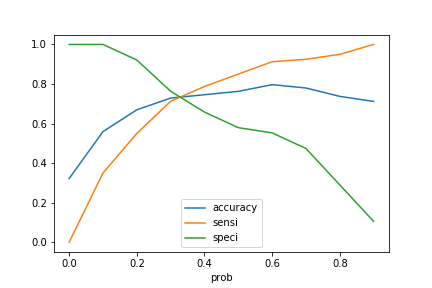# Evaluation-Sensitivity vs Specificity

## Sensitivity vs Specificity

This section explains the difference between two evaluation criteria, Sensitivity vs Specificity.

The below table shows the comparison between the actual value and predicted value.

Predicted
Actual No(Non-Diabetic) Yes(Diabetic)
No(Non-Diabetic) 68 12
Yes(Diabetic) 16 22

This table explains that the actual count of non-diabetic is 80 (68 + 12), whereas our model predicted 68 correctly. Similarly, the model predicted 22 correctly out of 38(16 + 22) for diabetic patients. So the accuracy of the model will be as below.In the given example accuracy = 76%.However, accuracy is not the only metric to evaluate the model. Now Let’s understand the difference between sensitivity vs specificity.

### Sensitivity

Sensitivity is the accuracy of correctly predicting diabetes. In other terms, you can say the accuracy of Yes.In the given example Sensitivity = 57%. In other words, the accuracy of being diabetic is 57%.

### Specificity

Specificity is the accuracy of correctly predicting non-diabetic or Nos.In the given example Specificity = 85%.

The above expressions can also be defined as below.

Predicted
Actual No(Non-Diabetic) Yes(Diabetic)
No(Non-Diabetic) True Positive False Positive
Yes(Diabetic) False Negative True NegativeNow let’s find out sensitivity and specificity for the diabetes dataset.

```
import pandas as pd
import numpy as np
from sklearn.model_selection import train_test_split

df = pima[['No_Times_Pregnant', 'Plasma_Glucose', 'Diastolic_BP', 'Triceps','Insulin', 'BMI', 'Age']]
normalized_df=(df-df.mean())/df.std()
pima = pima.drop(['No_Times_Pregnant', 'Plasma_Glucose', 'Diastolic_BP', 'Triceps','Insulin', 'BMI', 'Age'], 1)
pima = pd.concat([pima,normalized_df],axis=1)

X = pima.drop(['Diabetes'],axis=1)
y = pima['Diabetes']
X_train, X_test, y_train, y_test = train_test_split(X, y, train_size=0.7, test_size=0.3, random_state=100)
#Now fit the model.
from sklearn.linear_model import LogisticRegression
from sklearn import metrics
logsk = LogisticRegression()
logsk.fit(X_train, y_train)```

Predict probabilities.

``` y_pred = logsk.predict_proba(X_test)
y_pred_df = pd.DataFrame(y_pred)
y_pred_1 = y_pred_df.iloc[:,]

Output:

``````	1
0 	0.849983
1 	0.156658
2 	0.384572
3 	0.350269
4 	0.044309``````

Mark predicted value as 0 or 1 based on probability value.

```
y_test_df = pd.DataFrame(y_test)
y_test_df['CustID'] = y_test_df.index
y_pred_1.reset_index(drop=True, inplace=True)
y_test_df.reset_index(drop=True, inplace=True)
y_pred_final = pd.concat([y_test_df,y_pred_1],axis=1)
y_pred_final= y_pred_final.rename(columns={ 1 : 'diabetes_Prob'})
y_pred_final = y_pred_final.reindex_axis(['CustID','diabetes_Prob'], axis=1)
y_pred_final['predicted'] = y_pred_final.diabetes_Prob.map( lambda x: 1 if x > 0.5 else 0)

Evaluate the model.

```
from sklearn import metrics
confusion = metrics.confusion_matrix( y_test, y_pred_final.predicted )
confusion
```

Output: Confusion matrix

``````array([[68, 12],
[16, 22]])``````

Find overall accuracy.

` metrics.accuracy_score( y_test, y_pred_final.predicted)`

Output:

`0.7627118644067796`

Calculate both the metric and verify the values of sensitivity vs specificity.

```
TP = confusion[0,0] # true positive
TN = confusion[1,1] # true negatives
FP = confusion[0,1] # false positives
FN = confusion[1,0] # false negatives

# Let us calculate sensitivity and specificity
print("sensitivity ",TP / float(TP+FN))
print("specificity ",TN / float(TN+FP))
```

Output:

sensitivity 0.8095238095238095
specificity 0.6470588235294118

### The optimal cutoff between sensitivity and specificity

In the above calculation of Sensitivity vs Specificity, You can notice, though accuracy is high, sensitivity is very low, which is around 57%. If I want to focus more on the people who are suffering from diabetes, then in such case, the model will perform very badly. Based on business requirements, you may have to decide the cutoff point, whether to have a high sensitivity or high specificity. Though most of the time, we need to find an optimal point where sensitivity and specificity perform fairly well.

Let’s create columns with different probability cutoffs.

```
numbers = [float(x)/10 for x in range(10)]
for i in numbers:
y_pred_final[i]= y_pred_final.diabetes_Prob.map( lambda x: 1 if x > i else 0)

Output:

CustID diabetes_Prob predicted 0.0 0.1 0.2 0.3 0.4 0.5 0.6 0.7 0.8 0.9
0 124 0.849983 1 1 1 1 1 1 1 1 1 1 0
1 140 0.156658 0 1 1 0 0 0 0 0 0 0 0
2 276 0.384572 0 1 1 1 1 0 0 0 0 0 0
3 252 0.350269 0 1 1 1 1 0 0 0 0 0 0
4 326 0.044309 0 1 0 0 0 0 0 0 0 0 0

Now let’s calculate accuracy sensitivity and specificity for various probability cutoffs.

```cutoff_df = pd.DataFrame( columns = ['prob','accuracy','sensi','speci'])
from sklearn.metrics import confusion_matrix
num = [0.0,0.1,0.2,0.3,0.4,0.5,0.6,0.7,0.8,0.9]
for i in num:
cm1 = metrics.confusion_matrix( y_test, y_pred_final[i]  )
#print(cm1)
total1=sum(sum(cm1))
#print(total1)
accuracy = (cm1[0,0]+cm1[1,1])/total1
sensi = cm1[0,0]/(cm1[0,0]+cm1[0,1])
speci = cm1[1,1]/(cm1[1,0]+cm1[1,1])
cutoff_df.loc[i] =[ i ,accuracy,sensi,speci]
print(cutoff_df)
```

Output:

``````prob  accuracy   sensi     speci
0.0   0.0  0.322034  0.0000  1.000000
0.1   0.1  0.559322  0.3500  1.000000
0.2   0.2  0.669492  0.5500  0.921053
0.3   0.3  0.728814  0.7125  0.763158
0.4   0.4  0.745763  0.7875  0.657895
0.5   0.5  0.762712  0.8500  0.578947
0.6   0.6  0.796610  0.9125  0.552632
0.7   0.7  0.779661  0.9250  0.473684
0.8   0.8  0.737288  0.9500  0.289474
0.9   0.9  0.711864  1.0000  0.105263``````

Let’s plot accuracy sensitivity and specificity for various probabilities.

` cutoff_df.plot.line(x='prob', y=['accuracy','sensi','speci']) `